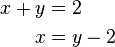# Frequently asked questions - Math

< Faq‎ | Math

Other languages:
čeština • ‎dansk • ‎English • ‎français • ‎italiano • ‎русский

## How do I align my equations at the equal sign?

Normally Math centers each line of a formula. To align each line at an equal sign you can use matrix, as shown in the following example.

Display Markup\begin{align} x + y &= 2 \\ x &= y-2 \end{align} matrix { alignr x+y # {}={} # alignl 2 ## alignr x # {}={} # alignl 2-y }

The empty braces around the equal sign are necessary because = is a binary operator that needs an expression on each side.

The space around the = can be reduced by changing the spacing between columns of the matrix:

• Select Format ▸ Spacing.
• Click [Category] and choose Matrices from the drop down menu.
• Enter 0% for Column spacing.

Sometimes you need to "reserve" some space on the left of the equal sign in multi-line equations. Use phantom as shown below.

Display Markup\begin{align} 3(x+4)-2(x-1) &= 3 x+12-(2 x-2) \\ &= 3 x+12-2 x+2 \\ &= x+14 \end{align} ""3(x+4)-2(x-1)=3 x+12-(2 x-2) newline
""phantom {3(x+4)-2(x-1)}=3 x+12-2 x+2 newline
""phantom {3(x+4)-2(x-1)}=x+14

The phantom markup indicates that there should be a space the same length as "3(x+4)-2(x-1)". This results in the equal signs lining up properly.

Note: The "" can also be replaced with alignl.